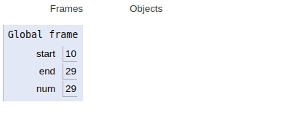# Python program to print all even numbers in a range

In this article, we will learn about the solution and approach to solve the given problem statement.

## Problem statement

Given a range, we need to print all the even numbers in the given range.

The brute-force approach is discussed below −

Here we apply a range-based for loop which provides all the integers available in the input interval.

After this, a check condition for even numbers is applied to filter all the odd numbers.

This approach takes O(n) + constant time of comparison.

Now let’s see the implementation below −

## Example

start, end = 10, 29
# iteration
for num in range(start, end + 1):
# check
if num % 2 == 0:
print(num, end = " ")

## Output

10 12 14 16 18 20 22 24 26 28

All the variables and functions are declared in a global frame as shown in the figure below.